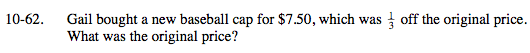### Home > MC2 > Chapter 10 > Lesson 10.1.5 > Problem10-62

10-62.$\text{If the new baseball cap is }\frac{1}{3}\text{ off the original price, then it must be }\frac{2}{3} \text{ of the original price.}$

Write an equation to represent the new cap's price as a fraction of the old cap's original price.

$(7.50) = \frac{2}{3} x$

x = \$11.25